Geometry problems (21..29)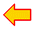problems 11..20

21.We see a rectangle and within two squares with areas 25 and 24.

calculate the size of the yellow colored area.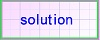22.
find angle x in the figure below.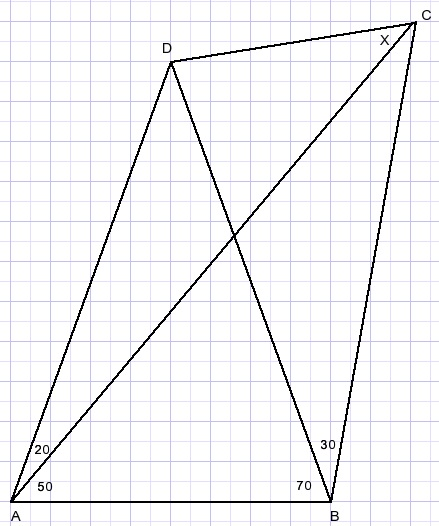23.
Calculate the radius of the small red circle in figure below.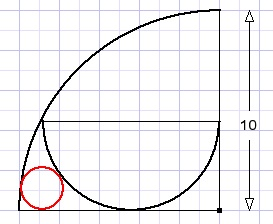24.
Calculate angle x in picture below.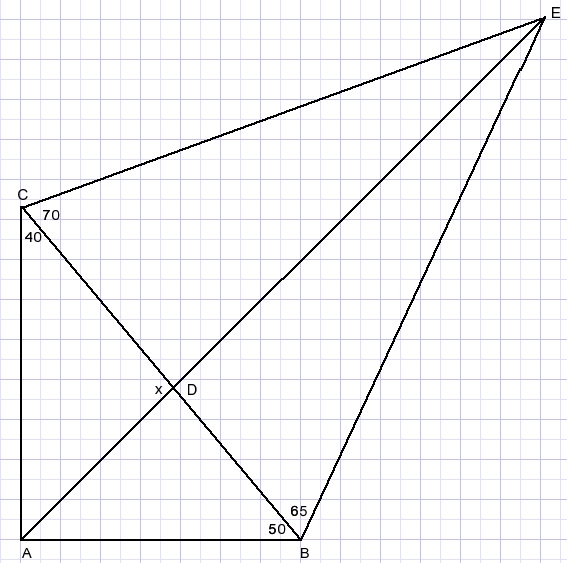25.
Calculate angel x in picture below.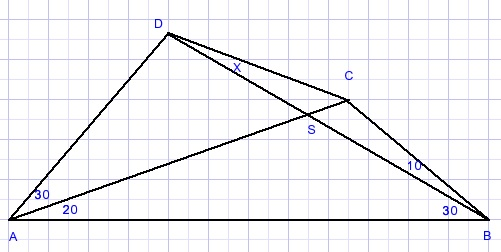26.
Calculate x in the picture below: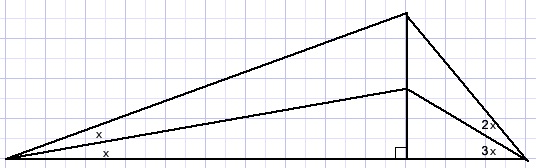27.
Calculate angle x in the picture below.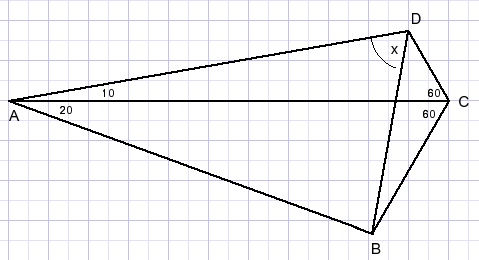28.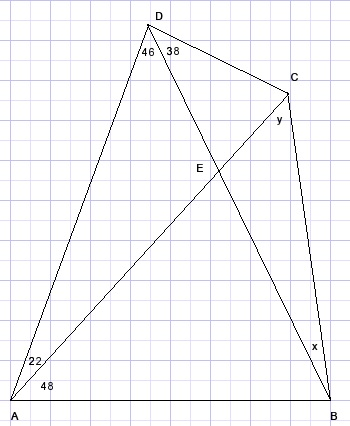Calculate angles x and y.29.
Calculate angle x: